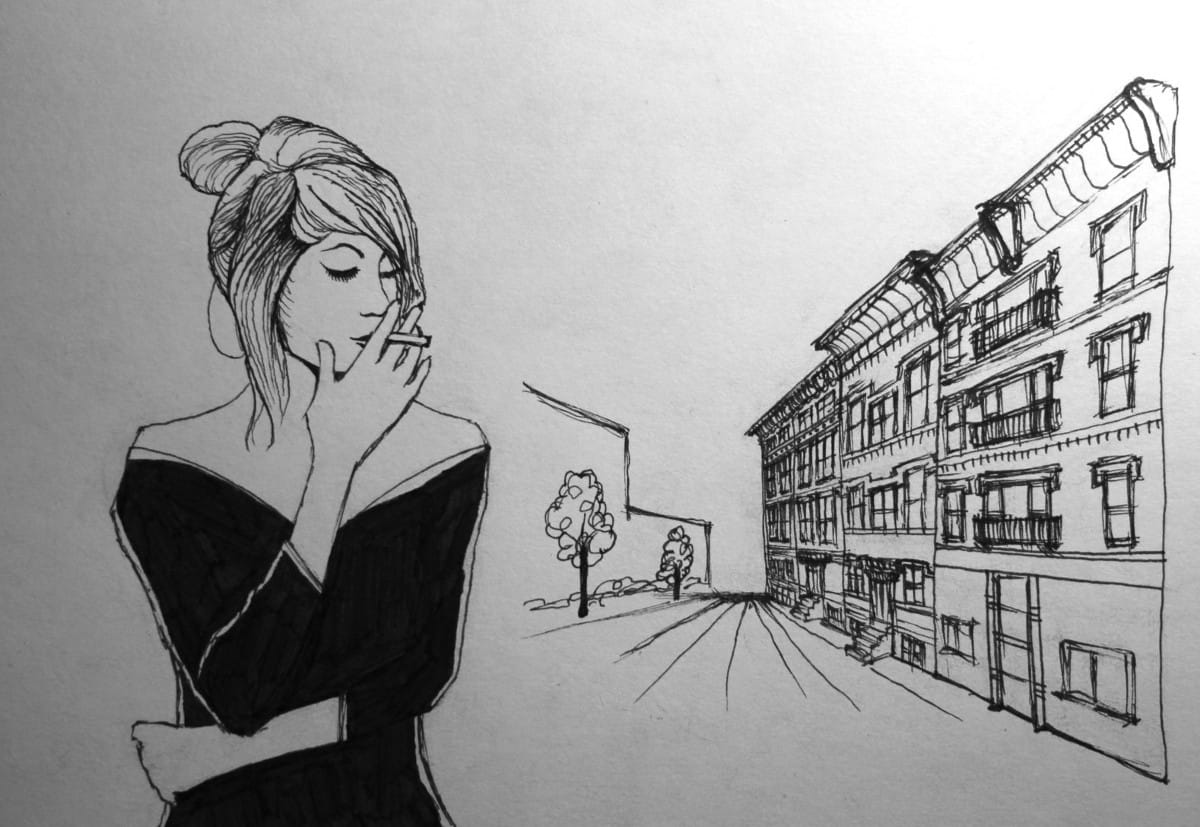# Sketch: Peonies

Thing practiced: drawing

Tools used: Prismacolor pencils, solvent, drawing paper

Haven’t posted for a while – it’s been busy, and finding a whole free hour any given day seems ambitious. I finally bought some colored pencils that aren’t meant for preschoolers, though, so I had to try them out.# Sketch: Valentine

Thing practiced: drawing

Tools used: sketch paper, colored pencils, pencil, eraser# Project Euler: #112-113, Bouncy numbers

Thing practiced: math

Tool used: Sublime Text 2

If no digit in a number is exceeded by the digit to its left it’s called an increasing number – e.g. 134,468. Similarly if no digit is exceeded by the digit to its right it’s called a decreasing number – e.g. 66,420. We’ll call a positive integer that is neither increasing nor decreasing a “bouncy” number – e.g. 155,349.

Clearly there cannot be any bouncy numbers below 100. In fact, the least number for which the proportion of bouncy numbers reaches 50% is 538. By the time we reach 21,780 the proportion of bouncy numbers is 90%.

Find the least number for which the proportion of bouncy numbers is exactly 99%.

Solution to 112:

This seems easy enough to brute-force, so we’ll try that in Python:

This gives us the answer: 1,587,000. (Python took 2.8 s to run this on a MacBook Air; out of curiosity I tried it in C — 42 ms.)

Next, problem 113:

As n increases, the proportion of bouncy numbers below n increases such that there are only 12,951 numbers below 1,000,000 that are not bouncy and only 277,032 non-bouncy numbers below 1010.

How many numbers below a googol (10100) are not bouncy?

Solution to 113:

Let’s start with a simple question and expand from there – how many increasing numbers are there below 1000?

Well, there are 3 digits in any number below 1000 (if we consider e.g. 012 to be 12), and for a number to be increasing each digit must be no lower than the digit to its left. Let’s label the digits in a 3-digit number A, B, and C. The leftmost digit A can be any of the digits 0-9; the middle digit B can be any of the digits from A-9; and the rightmost digit C can be any of the digits from B-9. That means that there are 12 potential digits to choose the 3 digits of our number from – the 10 digits 0-9, plus 2 potential duplicates if A = B or B = C.

The number of increasing numbers below 1000, then, is just (12 choose 3) = 220, minus 1 because we don’t want to count 000. Likewise, the number of increasing numbers below a googol is

$${109 \choose 100} - 1 \tag{increasing}$$

Decreasing numbers are similar, except that we can’t just ignore leading zeroes as we’d done with increasing numbers – for example, 072 is in fact a decreasing number, but wouldn’t be caught by the (12 choose 3) we used earlier. So, how many decreasing numbers are there below 1000?

If we label the digits in a 3-digit decreasing number D, E, and F, then the leftmost digit D can be any of the digits 0-9; the middle digit E can be any of the digits 0-D, or, if D = 0, any of the digits 0-9; and the rightmost digit F can be any of the digits 0-E, or, if D = E = 0, any of the digits 0-9. That means that there are 13 potential digits to choose the 3 digits of our number from – the 10 digits 0-9, plus 2 potential duplicates if D = E or E = F, plus 1 for potential leading zero(es).

The number of decreasing numbers below 1000, then, is (13 choose 3) = 286, minus 4 because 000 will occur 4 times (one time as before, plus once for each digit). Analogously, the number of decreasing numbers below a googol is

$${110 \choose 100} - 101 \tag{decreasing}$$

Almost there! In order to find all the non-bouncy numbers, we just need to add the increasing numbers to the decreasing numbers. However, some numbers are increasing and decreasing – e.g. 888. How many of these monotonic numbers are there? Well, for each power of 10 there are 9 potential monotonic numbers – e.g. we have 1, 2, ..., 9, 11, 22, ..., 99, 111, 222, ..., 999, etc. Since we have 100 digits, we have:

$$9 \times 100 \tag{duplicates}$$

Thus, the total number of non-bouncy numbers below a googol should be:

$$\left({109 \choose 100} - 1\right) + \left({110 \choose 100} - 101\right) - (9 \times 100) \tag{total}$$

This gives us a final solution of 51,161,058,134,250.

# Sketches: Momo

Thing practiced: drawing

Tools used: index cards, 4B pencil, eraser, pen